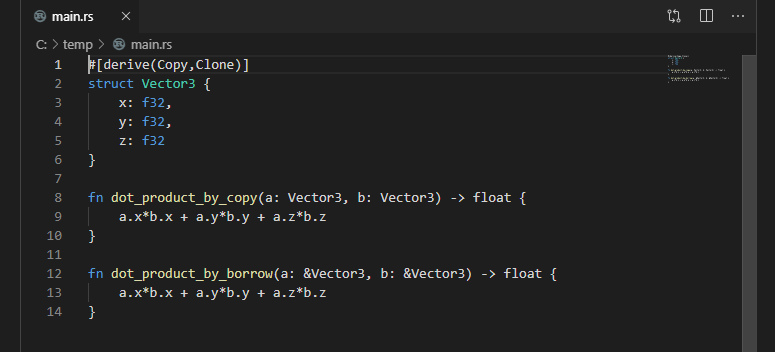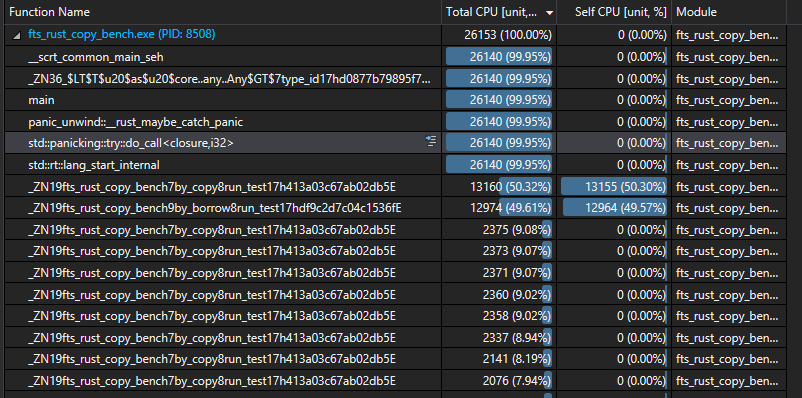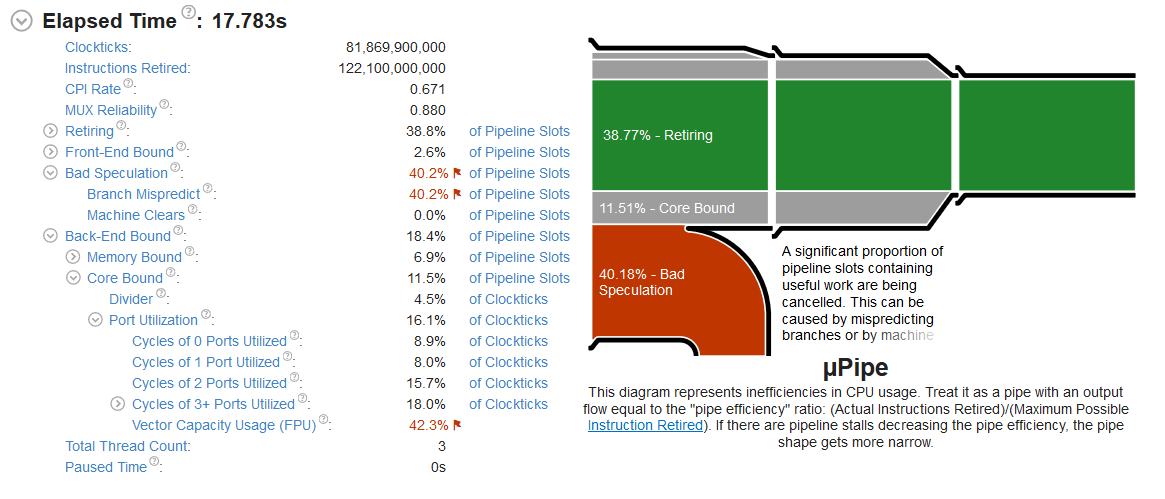Home

## 小的Rust结构类型,应使用复制还是借用传递?(译)# 对于小的 Rust 结构类型，应使用复制（copy）还是借用(borrow)传递？

2019 年 8 月 26 日 ❤️ 原文

``````struct Vector3 {
x: f32,
y: f32,
z: f32
}

fn dot_product_by_copy(a: Vector3, b: Vector3) -> float {
a.x*b.x + a.y*b.y + a.z*b.z
}

fn dot_product_by_borrow(a: &Vector3, b: &Vector3) -> float {
a.x*b.x + a.y*b.y + a.z*b.z
}
``````

## 为什么这个问题是重要的呢

### 人体工程学

``````struct Vector3 {
float x;
float y;
float z;
};

float dot_product(Vector3 const& a, Vector3 const& b) {
return a.x*b.x + a.y*b.y + a.z*b.z
}

Rust的问题是人体工程学。当以复制传递时，您可以将数学运算清晰而简单地组合在一起。

```rust
fn do_math(p1: Vector3, p2: Vector3, d1: Vector3, d2: Vector3, s: f32, t: f32) -> f32 {
let a = p1 + s*d1;
let b = p2 + s*d2;
dot_product(b - a, b - a)
}
``````

``````fn do_math(p1: &Vector3, p2: &Vector3, d1: &Vector3, d2: &Vector3, s: f32, t: f32) -> f32 {
let a = p1 + &(&d1*s);
let b = p2 + &(&d2*t);
let result = dot_product(&(&b - &a), &(&b - &a));
}
``````

## 建立基准

Twitter，Reddit 或 StackOverflow 都没有得到很好的答案。我检查了像 nalgebra（借用）和 cgmath（按值）的流行箱子，发现两种方式都很常见。

``````let num_shapes = 4000;
for cycle in 0...5 {
let (spheres, capsules, segments, triangles) = generate_shapes(num_shapes);
for run in 0..5 {
for (a,b) in collision_pairs {
test_by_copy(a,b)
}
for pair in collision_pairs {
test_by_borrow(&a, &b)
}
}
}
``````

``````  Rust
f32 by-copy:   7,109
f32 by-borrow: 7,172 (0.88% slower)

f64 by-copy:   9,642
f64 by-borrow: 9,601 (0.42% faster)
``````

## 随我来到 C ++ Rabbit Hole

``````  C++
f32 by-copy:   14,526
f32 by-borrow: 13,880 (4.5% faster)

f64 by-copy:   13,439
f64 by-borrow: 13,942 (3.8% slower)
``````

1. `double`(f64)按值传递是`float`按值传递
2. C ++ `float`比 Rust 的`f32`慢了两倍

### 内联C ++ 分析Rust 分析

``````  C++ w/ inlining
f32 by-copy:   12,688
f32 by-borrow: 12,108 (4.5% faster)

f64 by-copy:   11,860
f64 by-borrow: 11,967 (0.9% slower)
``````

### 别名

``````// Rust
fn closest_pt_segment_segment(p1: Vector3, q1: Vector3, p2: Vector3, q2: Vector3)
-> (T, T, T, Vector3, Vector3)
{
// Do math
}
``````
``````// C++
float closest_pt_segment_segment(
Vector3 p1, Vector3 q1, Vector3 p2, Vector3 q2,
float& s, float& t, Vector3& c1, Vector3& c2)
{
// Do math
}
``````

``````void run_test(
vector<TSphere> const& _spheres,
vector<TCapsule> const& _capsules,
vector<TSegment> const& _segments,
vector<TTriangle> const& _triangles,
int64_t& num_overlaps,
int64_t& milliseconds)
{
// perform overlaps
}
``````

``````  C++ w/ inlining, tuples
f32 by-copy:   12,863
f32 by-borrow: 11,555 (10.17% faster)

f64 by-copy:   11,832
f64 by-borrow: 11,524 (2.60% faster)
``````

## 编译标志（Compile Flags）

• 代码速度优先（/Ot）
• 禁用异常
• 高级 Vector 扩展 2（/arch:AVX2）
• 浮点模式:快速（/fp:fast）
• 启用浮点异常:否（/fp:except-）
• 禁用安全检查 /GS-
• 控制流防护: No

``````  C++ w/ inlining, tuples, no C++ exceptions
f32 by-copy:   11,651
f32 by-borrow: 10,455 (10.27% faster)

f64 by-copy:   10,866
f64 by-borrow: 10,467 (3.67% faster)
``````

## 更深入``````  Rust f32    42.3%
Rust f64    32.7%
C++  float  12.5%
C++  double 25.0%
``````

Yowza！无论出于何种原因，我都不知道究竟是什么，Rust 在利用 CPU 的浮点向量方面效率更高。差异是巨大的！Rust`f32`效率几乎是 C ++ `float`的 3.5 倍。并且，出于某种原因，C ++ `double``float`效率的两倍！🤷♂️

## 复制与借用传递

1. “小”的定义未经测试且未知。
2. 鉴于较少的自动 Vector 化，较少的数学结构可能不会起作用。
3. 高性能复制代码可能需要内联，和要对编译器相当信任。😱
4. 包括在另一个箱子中实现的小结构是未经测试的。
5. 综合基准并不反映现实世界。常记测量！

FullProjects.zip

## Clang

``````  C++ (Clang) w/ inlining, tuples, no C++ exceptions
f32 by-copy:   10416
f32 by-borrow: 10072 (3.30% faster)

f64 by-copy:   10520
f64 by-borrow: 11335 (7.47% slower)
``````